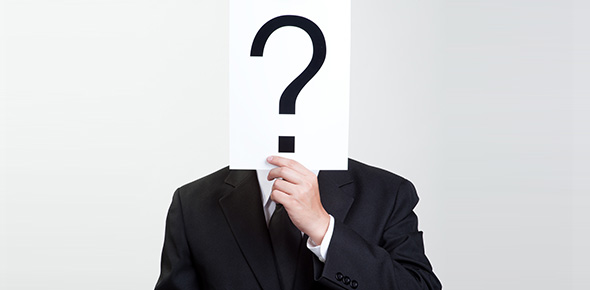# Post Scientific Concepts 4b*

24 Questions | Total Attempts: 38Settings.

• 1.
A science teacher asked her students to make a chart identifying the physical properties of each object below:Which of the following would be best to use for this chart of physical properties?
• A.

Age, color, length, mass

• B.

Hardness, mass, name, odor

• C.

length, shape, name, texture

• D.

Color, hardness, mass, length

• 2.
The characteristic of an object that has mass and takes up space is:
• A.

Volume

• B.

Mass

• C.

Matter

• D.

Weight

• 3.
One of the illustrations below is used to measure the amount of matter that  makes up an object :
• A.

Option 1

• B.

Option 2

• C.

Option 3

• D.

Option 4

• 4.
The parts of an electromagnet are:
• A.

Battery, battery holder, nail, wire

• B.

Battery, battery holder, bulb, wire

• C.

Battery, battery holder, nail, bulb

• D.

Battery, battery holder, switch, wire

• 5.
The two phases of matter in the illustration below are:
• A.

Solid and liquid

• B.

Solid and gas

• C.

Liquid and gas

• D.

Liquid and liquid

• 6.
What is electricity transformed into when you turn on a TV?
• A.

Light, motion, and sound

• B.

Light, sound, and heat

• C.

Light, heat, and motion

• D.

Motion, sound, and heat

• 7.
Property that compares an object’s mass to its volume is called:
• A.

Density

• B.

Property

• C.

Matter

• D.

Mass

• 8.
Which of the following colors represents the greatest density in the illustration below:
• A.

Red

• B.

Green

• C.

Yellow

• D.

Blue

• 9.
One of the following is a property of solid particles:
• A.

Tightly packed and held in place

• B.

Close to each other but they are not held tightly in place

• C.

Far apart

• D.

Take the shape of the container

• 10.
Which of the following will be attracted to the magnet:
• A.

Option 1

• B.

Option 2

• C.

Option 3

• D.

Option 4

• 11.
The  evaporation process in  water  cycle chart is number:
• A.

4

• B.

3

• C.

2

• D.

1

• 12.
The illustration that represents the liquid state of matter is:
• A.

Option 1

• B.

Option 2

• C.

Option 3

• D.

Option 4

• 13.
“Boiling egg” is a process that changes the egg from liquid to solid. This change is :
• A.

Melting

• B.

Physical change

• C.

Boiling

• D.

Chemical change

• 14.
An electric charge in motion is called:
• A.

Electric current

• B.

Insulator

• C.

Conductor

• D.

Chemical change

• 15.
Which one of the following circuits shows a complete circuit?
• A.

Option 1

• B.

Option 2

• C.

Option 3

• D.

Option 4

• 16.
Look carefully at the illustration below. What form of energy is created when a magnet moves near a wire?
• A.

Electrical energy

• B.

Sound energy

• C.

Light energy

• D.

Kinetic energy

• 17.
In the illustration below the number that points to the resistor is:
• A.

1

• B.

2

• C.

3

• D.

4

• 18.
Series circuit is a type of circuits that:
• A.

The current moves through it when there is a broken loop

• B.

Has two or more paths through which electric charges may flow

• C.

Has resistors but no source of energy

• D.

Electric charge can flow only in one circular path

• 19.
All magnets have two:
• A.

Sides

• B.

Names

• C.

Sounds

• D.

Poles

• 20.
Which letter of the following magnets represents “repel”:
• A.

A

• B.

B

• C.

C

• D.

D

• 21.
In the next illustration, if lamp 1 is removed from its holder, what will happen to lamp 2 :
• A.

It will stay working

• B.

It will not work

• C.

It will get dimmer

• D.

It will burn

• 22.
The best symbol  below that represents the resistor is:
• A.

Option 1

• B.

Option 2

• C.

Option 3

• D.

Option 4

• 23.
Ahmad needs to buy a light bulb which saves energy for his room. He found two types of them, the incandescent light bulb and the fluorescent light bulb. Use the following line graph to help Ahmad find that type of light bulbs.
• A.

Fluorescent

• B.

Incandescent

• C.

Electronic

• D.

Filament

• 24.
The circuit below is one type of circuits that are used at homes and schools :
• A.

Parallel circuit

• B.

Series circuit

• C.

New circuit

• D.

GeneratorBack to top